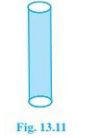# NCERT Solutions Class 9 Mathematics Solutions for Surface Areas and Volumes - Exercise 13.2 in Chapter 13 - Surface Areas and Volumes

A metal pipe is 77 cm long. The inner diameter of a cross-section is 4 cm, the outer diameter

being 4.4cm. (see fig. 13.11). Find its(i) inner curved surface area,

(ii) outer curved surface area

(iii) total surface area

(Assume π=22/7)

Let 𝑟1 and 𝑟2 Inner and outer radii of a cylindrical pipe

𝑟1 = 4/2 cm = 2cm

𝑟2 = 4.4/2 cm = 2.2 cm

Height of cylindrical pipe, h = length of cylindrical pipe = 77 cm

(i)the curved surface area of the outer surface of pipe = 2π 𝑟1 h

\begin{array}{l} =2 \times 22 / 7 \times 2 \times 77 \mathrm{cm}^{2} \\ =968 \mathrm{cm}^{2} \end{array}

(ii) curved surface area of outer surface of pipe = 2π 𝑟2 h

= 2 × 22/7 × 2.2 × 77 \mathrm{cm}^{2}

\begin{array}{l} =(22 \times 22 \times 2.2) \mathrm{cm}^{2} \\ =1064.8 \mathrm{cm}^{2} \end{array}

(iii) Total surface area of pipe = inner curved surface area + outer curved surface area + Area of

both circular ends of the pipe.

\begin{array}{l} =2 \pi r_{1} h+2 \pi r_{2} h+\left(r_{2}^{2}-r_{1}^{2}\right) \\ =9668+1064.8+2 \pi\left((2.2)^{2}-2^{2}\right) \\ =2031.8+5.28 \end{array}

2038.08 \mathrm{cm}^{2}

Therefore, the total surface area of the cylindrical pipe is 2038.08 \mathrm{cm}^{2}

Related Questions

Lido

Courses

Teachers

Book a Demo with us

Syllabus

Maths
CBSE
Maths
ICSE
Science
CBSE

Science
ICSE
English
CBSE
English
ICSE
Coding

Terms & Policies

Selina Question Bank

Maths
Physics
Biology

Allied Question Bank

Chemistry
Connect with us on social media!